Get instant live expert help with Excel or Google Sheets“My Excelchat expert helped me in less than 20 minutes, saving me what would have been 5 hours of work!”

#### Post your problem and you’ll get expert help in seconds.

Your message must be at least 40 characters
Our professional experts are available now. Your privacy is guaranteed.

# Excel Dynamic Lookup Table with INDIRECT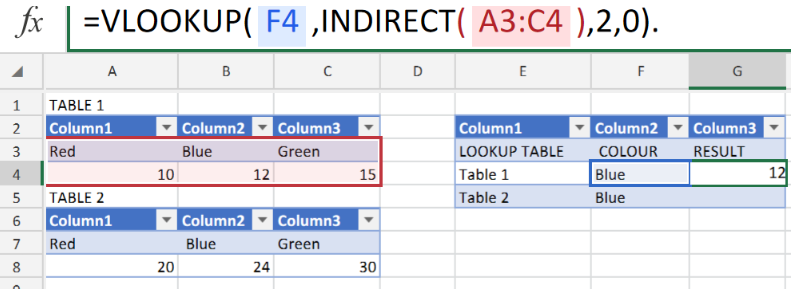Figure 1. of Dynamic Lookup Table with INDIRECT.

In order to apply a Dynamic Lookup Table in our Excel worksheet, we are going to utilize the Excel INDIRECT function together with a specific cell range inside of the VLOOKUP operation syntax. This tutorial will step through the process.

## Generic Formula

`=VLOOKUP(A1,INDIRECT("text"),column)`

This is essentially a VLOOKUP operation syntax. However, the INDIRECT operation is also applied to determine a valid table range.

How to use the VLOOKUP and INDIRECT Function in Excel.

Our purpose here is to provide an easy way to switch the ranges of our tables within a VLOOKUP Function.

We can achieve this by following 3 simple steps!

1. Collate our data values in the form of Tables in our Excel sheet.

Prepare separate Tables for data values and our dynamic Lookup Table, in the example illustrated below, we have created two named ranges/tables table 1=A3:C4 and table 2=A7:C8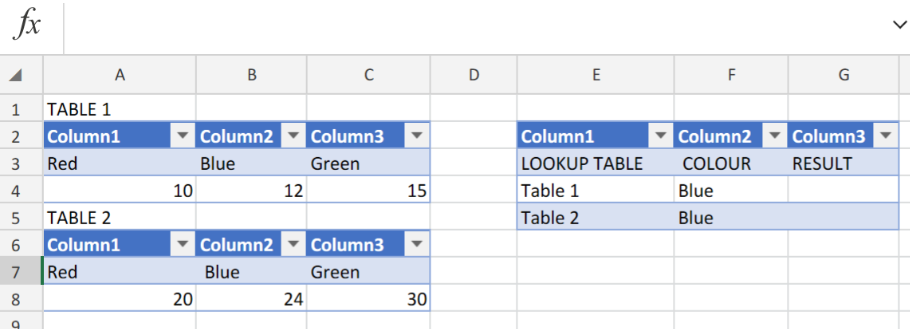Figure 2. of Dynamic Lookup Table in Excel.

1. Input the following formula syntax into the formula bar for cell G4;

`=VLOOKUP(F4,INDIRECT(A3:C4),2,0)`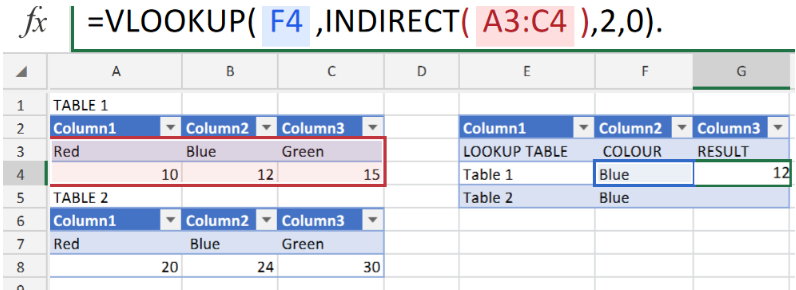Figure 3. of Dynamic Lookup Table with INDIRECT Function in Excel.

The INDIRECT function will select the table 1 cell range and resolves it with the table 2 cell range which is then determined by the VLOOKUP function

VLOOKUP executes the lookup operation and calculates our specified colour, blue as 12 in Table 1 of our worksheet illustration.

1. Copy and paste a  modified formula into cell G5 to get the result for Table 2. See example illustrated below;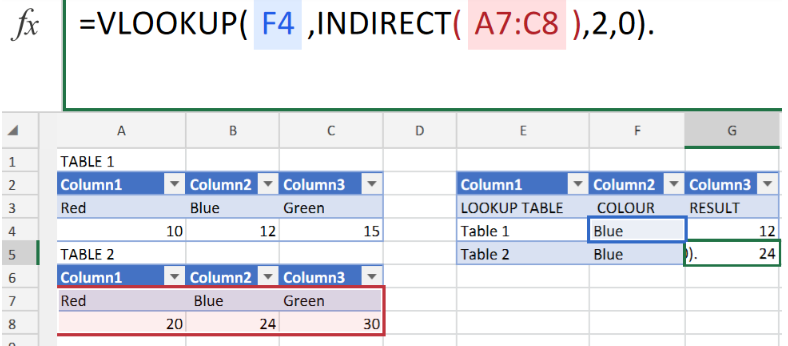Figure 4. of Dynamic Lookup Table with INDIRECT Function in Excel.

The objective of the Dynamic Lookup Table with INDIRECT Function is to provide us with an easy method of switching table ranges inside a Lookup function.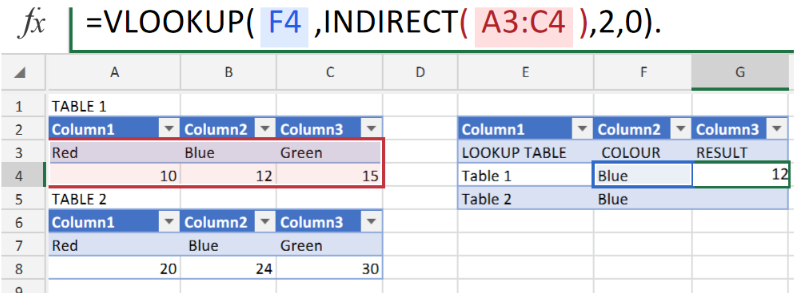Figure 5. of Final Result.

## Instant Connection to an Expert through our Excelchat Service:

Our live Excelchat Service is here for you. We have Excel Experts available 24/7 to answer any Excel questions you may have. Guaranteed connection within 30 seconds and a customized solution for you within 20 minutes.

Solution examplesI have a pivot table in which per order (on the rows) stands how much products they ordered per size (on the columns). I want to determine which combinations of quantities of sizes people order. And I want to count these combinations.
Solved by E. W. in 60 minsI have 500 numbers in column A with aproximatly 15digits. In column B I have 5 numbers with 6 digits Can a find a formula wich can transfer all numbers from A column wich start with some 6 digitsa from B column
Solved by V. L. in 32 minsI have a sheet with 3 columns. First column is a code for records in column B (has 563 records). What I need is a formula to show me if what is in column C (has 4400 records) is in column B and if it is to take the code for that record. Example: Column A(code corresponding to column B): 12, 14, 15, 19 Column B(names): asd, adf, ade, aqw Column C(names): akd, adf, ade, anb, wgs I need something that would say, if record in column C (for example adf) is matching record in column B (adf) return the code from column A (the code corresponding to that record in column B),if not return blank.
Solved by C. J. in 8 minsIf the data in A matches the data in C, then I need the data in E to be placed in B
Solved by X. W. in 40 minsI have a workbook with 2 sheets. First sheet has column A which is a device name, then columns B - infinity that have numeric "tags" for that device. Each device can have one or 20 "tags", tags are all numerical. Worksheet 2 has column A which is numeric value and B which is a human readable value of what the tag is. Question is, how do I replace all the tag numbers in worksheet 1 with the actual human readable values is sheet 2?
Solved by T. H. in 16 mins